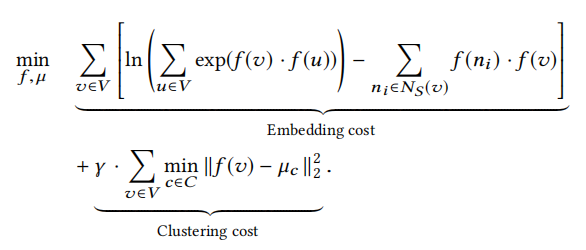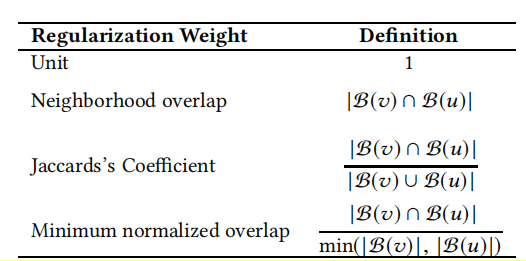# [论文阅读笔记] GEMSEC，Graph Embedding with Self Clustering

## 本文结构

1. 解决问题
2. 主要贡献
3. 算法原理
4. 参考文献

#### (2) 主要贡献

Contribution 1： 提出GEMSEC，一个基于序列的图表征模型，学习节点表征的同时进行节点的聚类。

Contribution 2： 引入平滑正则项来迫使具有高度重叠邻域的节点对有相似的节点表示。

#### (3) 算法原理

GEMSEC算法主要的框架还是遵循DeepWalk的算法框架，即随机游走生成语料库，再利用简单神经网络来训练节点表示向量。

• 对于随机游走部分，GEMSEC简单采用DeepWalk的一阶随机游走。
• 对于所使用的简单神经网络，原本DeepWalk采用的是以最大化窗口内节点共现概率为目标的Skip-Gram模型，而GEMSEC仅仅在Skip-Gram目标函数（窗口内节点的共现概率）的基础上加上了和聚类有关的目标函数，从而将表示向量学习目标和聚类目标联合在一起优化，得到更加适合聚类（簇的内聚程度高，簇间分明）的表示向量，在学习表示向量的同时也生成了节点的社区划分。$f-the\ mapping\ function\ (from\ node\ to\ embedding\ vector)$

$f(v)-the\ embedding\ vector\ of node\ v$

$N_{S}(v)-the\ collection\ of\ windows\ containing\ v$

$C-the\ set\ of\ communities$

$u_{c}-the\ vector\ of\ cluster\ center$

$\gamma-the\ weight\ coefficient\ of\ the\ clustering\ cost$$E_{S}-the\ edges\ within\ the\ windows$

$w(v,u)-the\ regularization\ weight\ of\ each\ pair\ (v,u)$

$\lambda-the\ regularization\ coefficient$#### (4) 参考文献

Rozemberczki B, Davies R, Sarkar R, et al. Gemsec: Graph embedding with self clustering[C]//Proceedings of the 2019 IEEE/ACM international conference on advances in social networks analysis and mining. 2019: 65-72.

posted @ 2020-10-15 09:17  沁泽  阅读(633)  评论(0编辑  收藏  举报